# Volume Calculator

## Find the Volume of a Cylinder, Volume of Sphere, Volume of Cuboid, Volume of a Cone, Volume of Cube, Volume of Pyramid and Volume of a Prism

HomeMath

Volume Calculator

=

## Volume of Cylinder Formula

### Volume of Solid Cylinder Formula

#### r is radius, h is height and Pi value is 3.14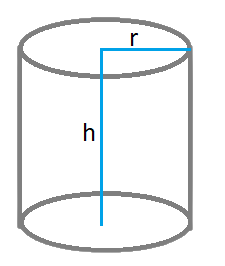### Volume of a Hollow Cylinder

#### R is outer radius, r is inner radius, h is height and Pi value is 3.14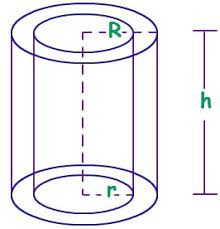## Volume of Sphere formula

### Formula to calculate Volume of a Sphere using Radius

#### r is radius and Pi value is 3.14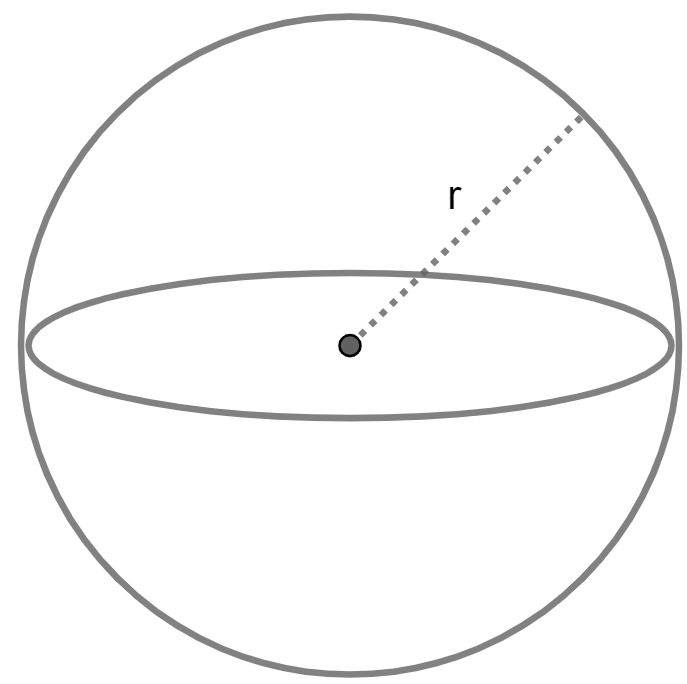## Volume of Hemisphere Formula

### Formula to calculate Volume of a Hemisphere using Radius

#### r is radius and Pi value is 3.14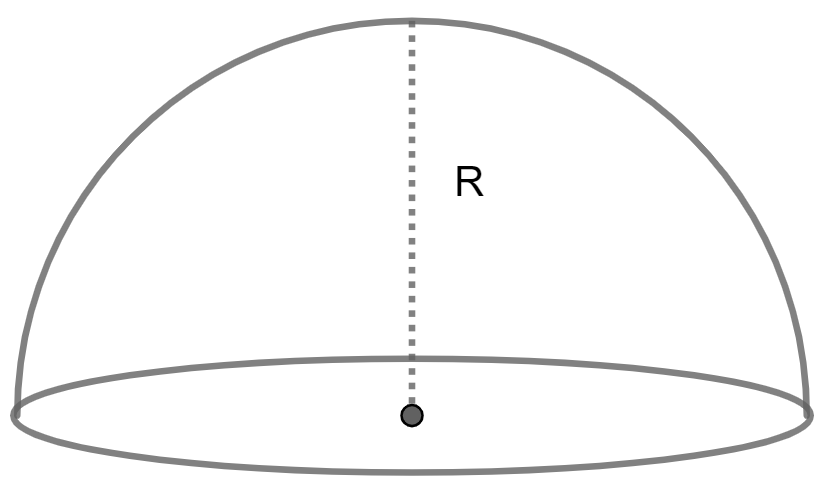## Volume of a Cone Formula

### Volume of a Cone Cylinder

#### r is radius, h is height and Pi value is 3.14## Volume of a Cube Formula

### Formula to calculate Volume of a Cube using Edge

#### a is edge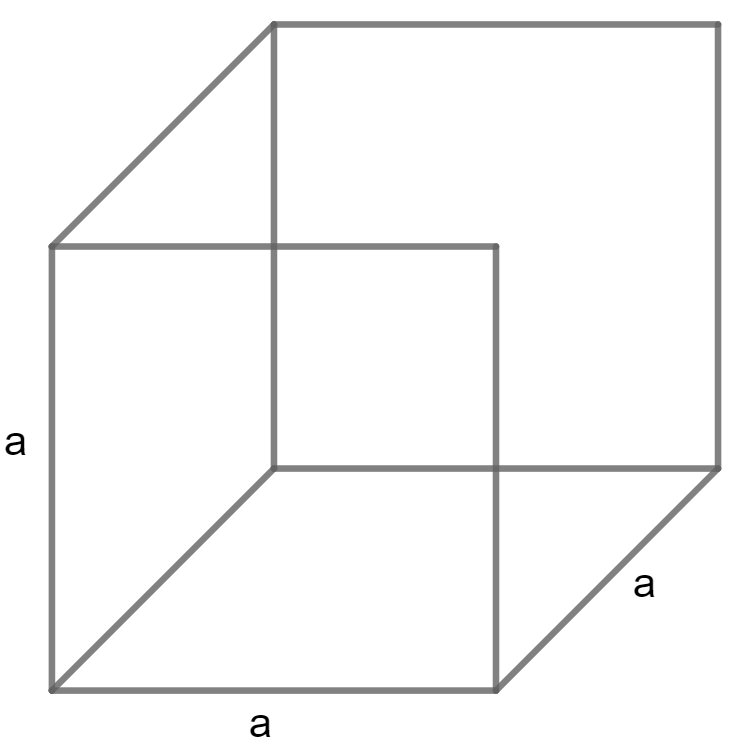## Volume of a Cuboid Formula

### Formula to calculate Volume of a Cuboid, Volume of a Rectangle using Length, Width and Height

#### L is Length, W is Width, and H is Height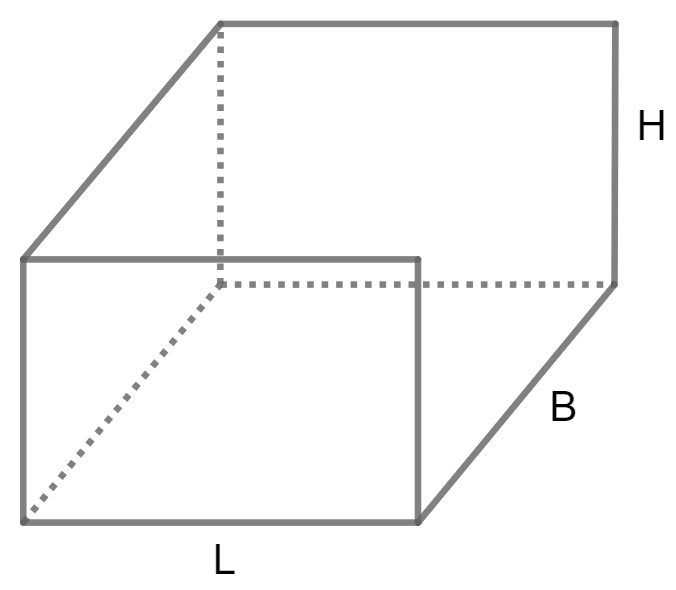## Pyramid Volume Formula

### Formula to calculate Volume of a Triangular Pyramid using Area and Height

#### A is Area and H is Height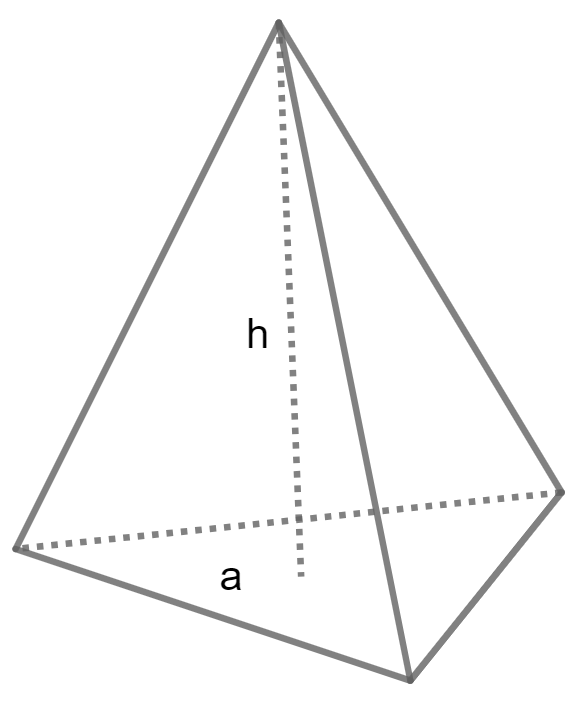### Formula to calculate Volume of a Square Pyramid using Base edge and Height

#### A is base edge and H is Height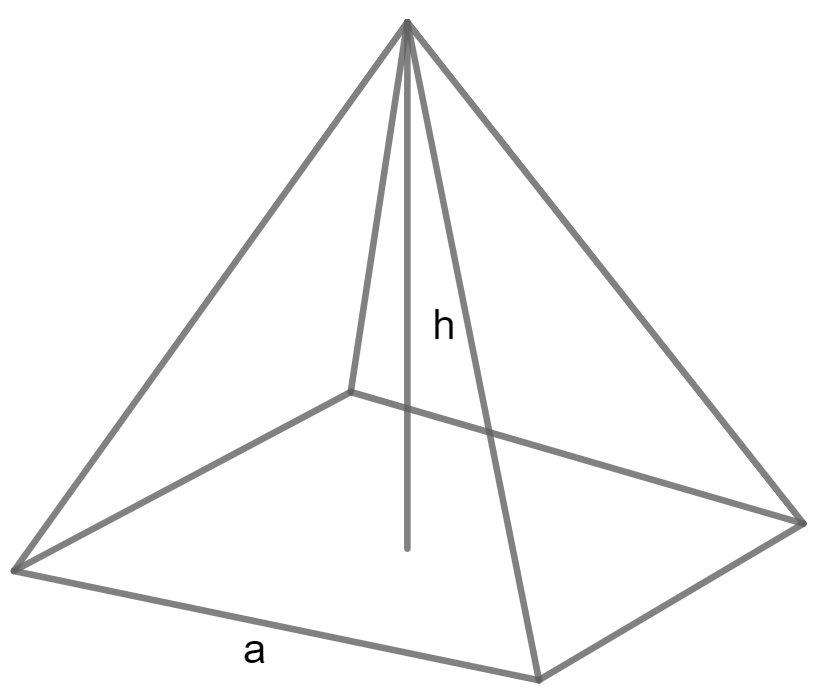### Formula to calculate Volume of a Pentagonal Pyramid using Base edge and Height

#### A is base edge and H is Height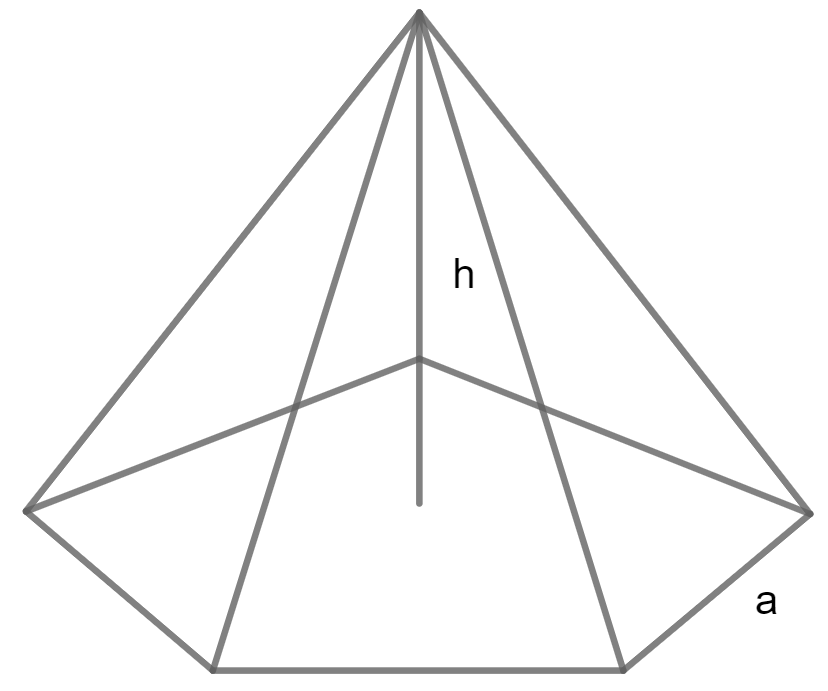### Formula to calculate Volume of a Rectangular Pyramid using Base length, Width and Height

#### L is base length, W is Width and H is Height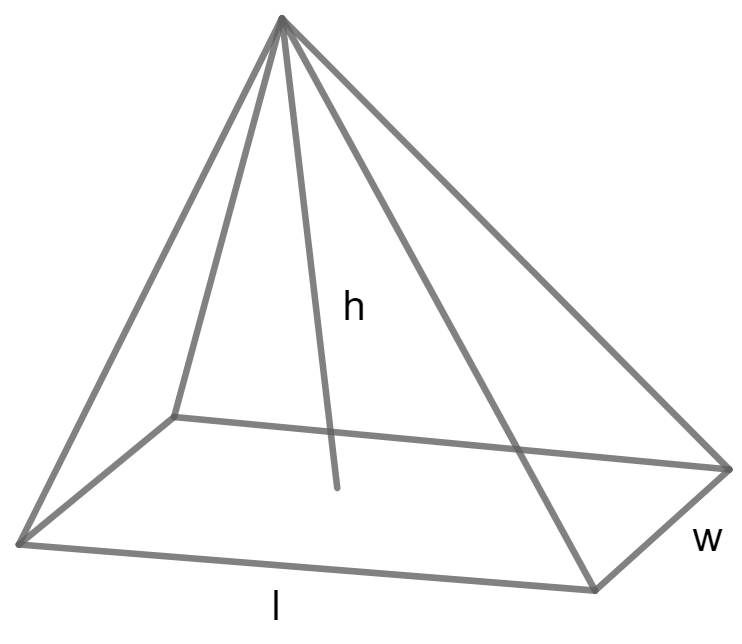## Volume of Prism Formula

### Formula to calculate Volume of a Triangular Prism using Base Edge, Length and Height

#### A is Base edge and H is Height and L is Length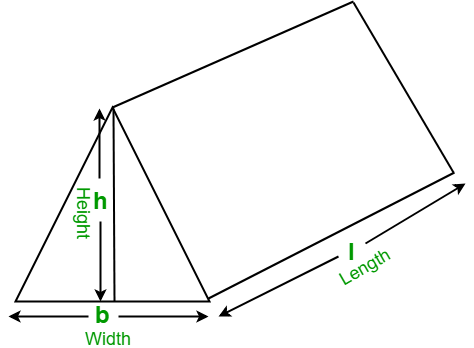### Formula to calculate Volume of a Square Prism using Base edge and Height

#### A is base edge and H is Height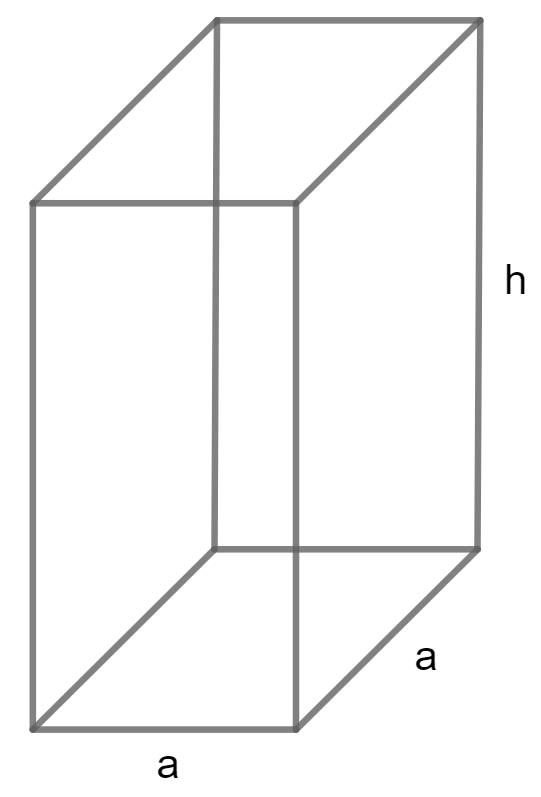### Formula to calculate Volume of a Rectangular Prism using Width, Length and Height

#### W is Width and L is Length and H is Height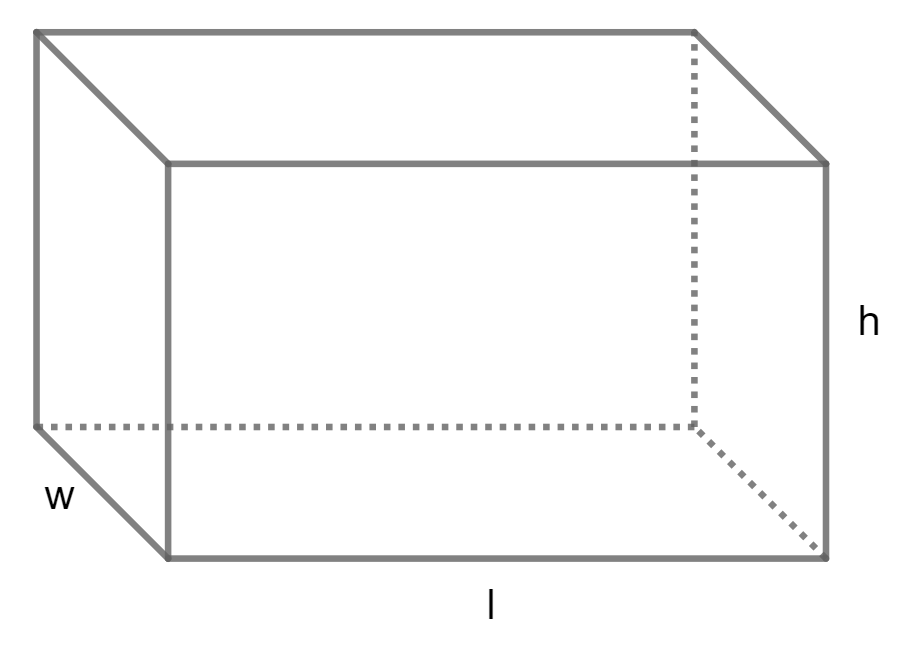### Formula to calculate Volume of a Pentagonal Prism using Base edge and Height

#### A is base edge and H is Height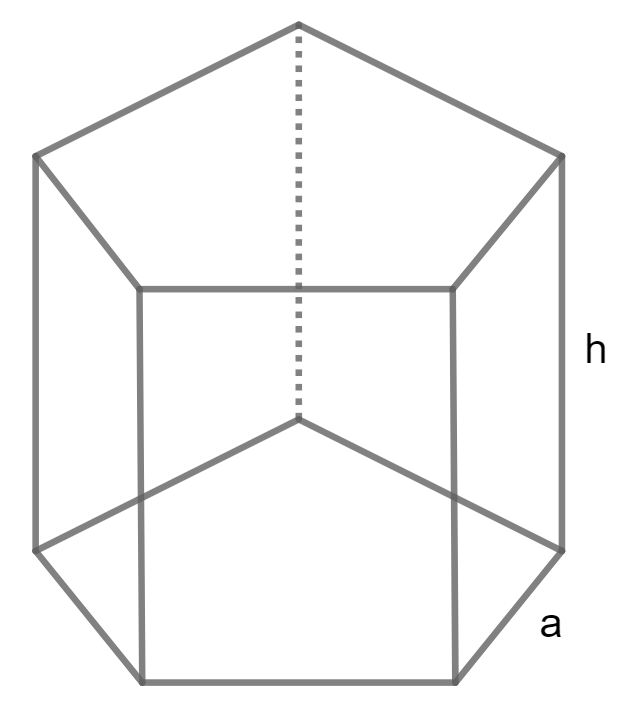### Formula to calculate Volume of a Hexagonal Prism using Base edge and Height

#### A is base edge and H is Height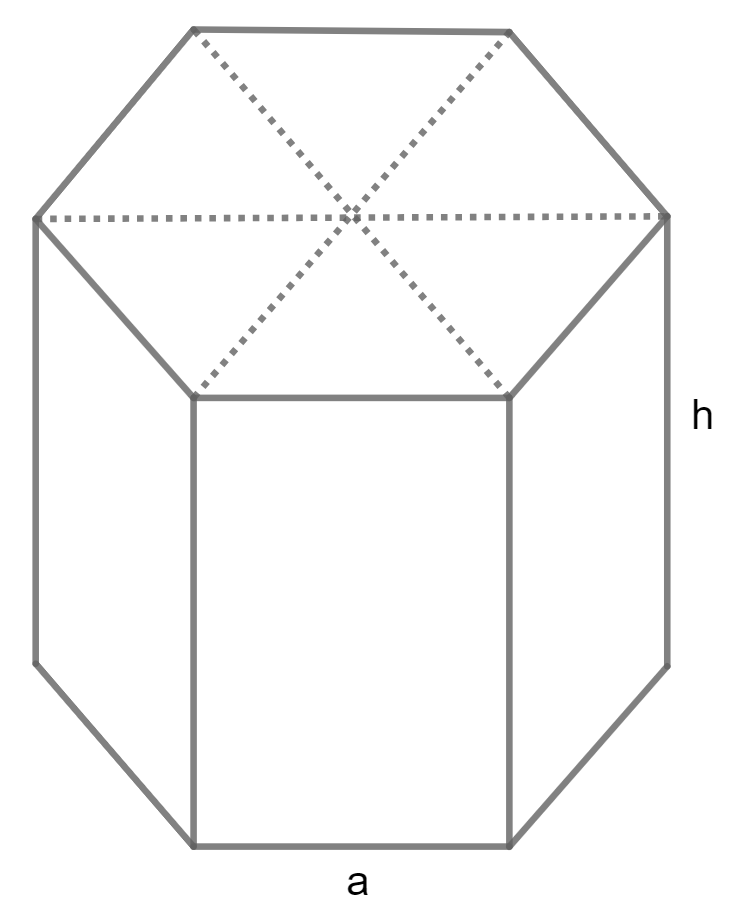## Ellipsoid Volume Formula

### Formula to calculate Volume of a Ellipsoid using Axes

#### A, B and C are axes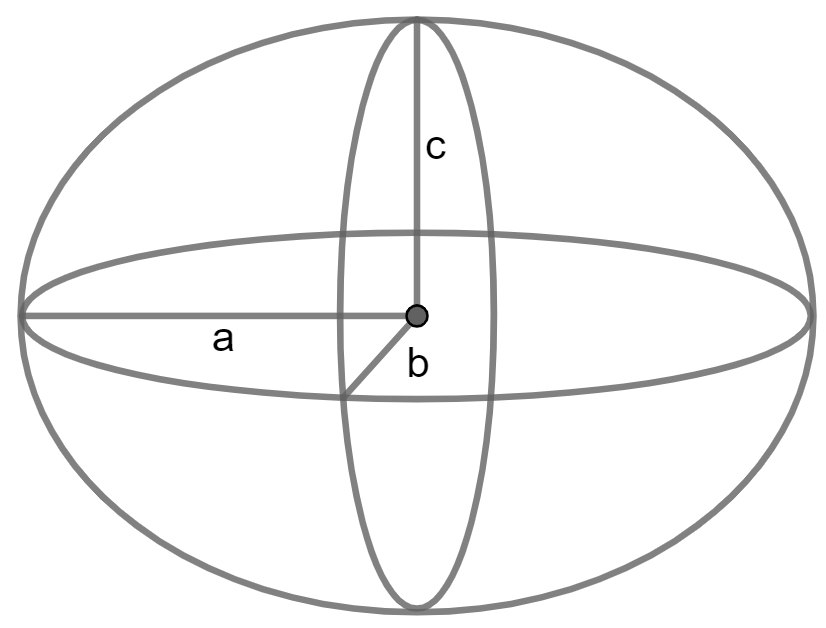## Tetrahedron Volume Formula

### Formula to calculate Volume of a Tetrahedron using Edge

#### A is Edge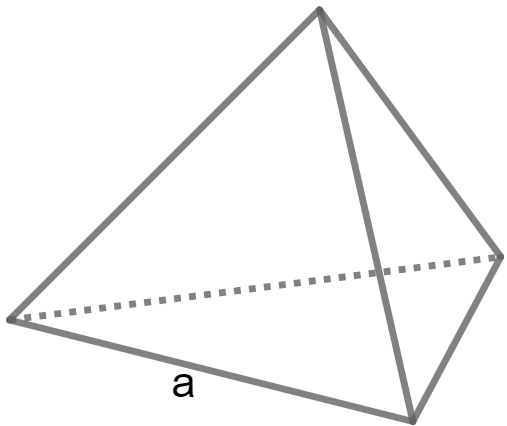## Hollow Cylinder Volume Formula

### Volume of a Hollow Cylinder

#### R is outer radius, r is inner radius, h is height and Pi value is 3.14## Spherical Cap Volume Formula

### Formula to calculate Volume of a Spherical Cap using Radius and Height

#### H is Height, R is Radius and Pi value is 3.14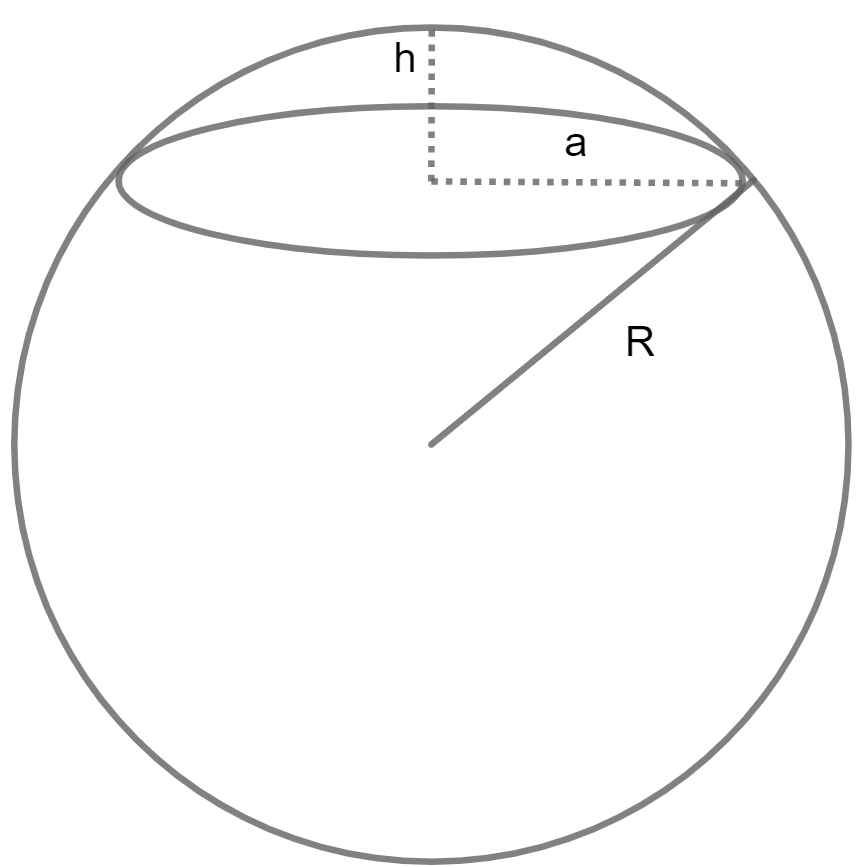## Frequently Asked Questions on Volume Calculator and Volume Formula